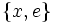# Characteristic not implies fully invariant

This article gives the statement and possibly, proof, of a non-implication relation between two subgroup properties. That is, it states that every subgroup satisfying the first subgroup property (i.e., characteristic subgroup) need not satisfy the second subgroup property (i.e., fully invariant subgroup)
View a complete list of subgroup property non-implications | View a complete list of subgroup property implications
Get more facts about characteristic subgroup|Get more facts about fully invariant subgroup
EXPLORE EXAMPLES YOURSELF: View examples of subgroups satisfying property characteristic subgroup but not fully invariant subgroup|View examples of subgroups satisfying property characteristic subgroup and fully invariant subgroup

## Statement

A characteristic subgroup of a group need not be a fully invariant subgroup.

## Definitions used

Term Definition
characteristic subgroup A subgroup$H$ of a group$G$ is termed a characteristic subgroup if for any automorphism$\sigma$ of$G$ and any$x \in H$,$\sigma(x) \in H$.
fully invariant subgroup A subgroup$H$ of a group$G$ is termed a fully invariant subgroup if for any endomorphism$\sigma$ of$G$ and any$x \in H$,$\sigma(x) \in H$.

## Related facts

### Some subgroup-defining functions yield fully invariant subgroups

All members of the derived series and the lower central series of a group are fully characteristic. This follows from the fact that they are all verbal subgroups (i.e., can be described as being generated by words of a certain form. Further information: Verbal subgroup, Verbal implies fully characteristic

## Proof

### The example of the dihedral group

Further information: Dihedral group:D8

In the dihedral group of order eight, there exists a cyclic characteristic subgroup of order four that is not fully characteristic. Specifically, if we have:$G := \langle a,x \mid a^4 = x^2 = e, xax^{-1} = a^{-1} \rangle$

then the subgroup generated by$a$ is characteristic. On the other hand, it is not fully characteristic: consider the homomorphism that sends both$a$ and$x$ to$x$. This is a retraction to the two-element subgroup$\{ x, e \}$, and under this retraction, the subgroup generated by$a$ is not invariant (since$a$ gets mapped to$x$).

### The example of the center, or a characteristic direct factor

If$G$ is a nontrivial centerless group and$A$ is an Abelian group isomorphic to a nontrivial Abelian subgroup$B$ of$G$, then we have:

•$A$ is characteristic in$G$: In fact,$A$ is the center of$G \times A$.
•$A$ is not fully characteristic in$G$: Consider the endomorphism with kernel$G$, mapping$A$ isomorphically to$B$.$A$ is not invariant under this endomorphism.